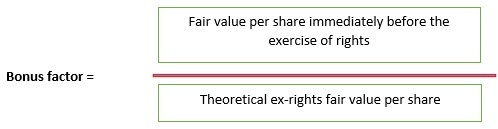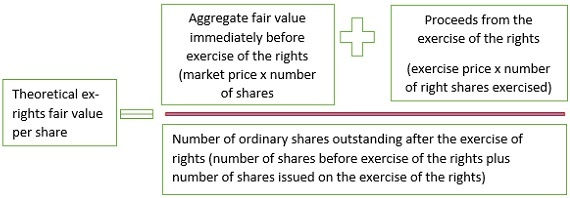# Ind AS 33: Impact of Rights Issue on calculation of Earnings Per Share

Earnings per share (‘EPS’), as the name suggests comprises of two factors:

1. The net profit or loss for the year/period attributable to the ordinary shareholders, and

2. The weighted average number of ordinary shares outstanding during the year/period.

While the first component of EPS is easy to determine, the second may pose various challenges especially when there is movement in the ordinary shares during the year/period for which EPS is to be calculated.

Issue of ordinary shares during the year can be in the form of fresh issue at fair value, bonus issue without any consideration received, issue of shares to the existing holders in lieu of dividends, right issue to the existing holders of ordinary shares at a price which is equal to or less than the fair value and so on and so forth.

This article specifically deals with impact of right issue at a price which is less than the fair value on calculation of EPS for the current as well as previous year.

In a right issue, existing ordinary shareholders can purchase additional ordinary shares generally on a pro rata basis and at price which is either equal to or less than the fair value of the shares.

For e.g.: Company A Ltd offers all its ordinary shareholders the right to purchase 1 ordinary share for every 4 ordinary shares held by them, at a discount of 25% on the fair value.

Now, if company A Ltd has 1000 ordinary shares and the fair value of its share is Rs. 10 per share, then the right issue will comprise of 250 ordinary shares at a price of Rs. 7.5 per share.

The difference between the issue price i.e. Rs. 7.5 and the fair value i.e. Rs. 10 amounting to Rs. 2.5 is the bonus element which needs to be considered in the calculation of the EPS.

When there is bonus element involved in any issue, Ind AS 33 requires retrospective adjustment to the weighted average number of ordinary shares.

This adjustment is called the ‘Bonus Factor’ and is calculated using the following formula:

Bonus factor =

Let’s understand the above components involved in the bonus factor formula:

Fair value per share immediately before the exercise of rights

This is the market price of the shares immediately before it becomes “ex-rights” or last day when the shares are traded together with the rights i.e. “cum-rights”2. Theoretical ex-rights fair value per share

This number is calculated using the following formula:Let us now understand this complex formula with the help of an easy illustration:

Taking forward our above illustration of Company A Ltd issuing 250 right issue at a discounted price of Rs. 7.5, the following are the additional information available:

• Market price on the last day when the shares are traded cum rights is Rs. 10
• A Ltd has 1000 ordinary shares prior to the right issue
• The last exercise date is 1 April 20X1
• All the rights are exercised i.e. A Ltd issued 250 ordinary shares on right issue
• A Ltd has a profit attributable to its ordinary shareholder in the year 20X0 of Rs. 200,000 and Rs. 250,000 in year 20X1
• The company follows calendar year for the purpose of its books of accounts

With these facts let us know calculate the EPS (for simplicity only basic EPS is considered in the computation)

Step 1: Calculate the numerator i.e. net profit attributable to the ordinary shareholders for EPS:

As discussed above the first factor or the numerator to be considered for EPS is the net profit attributable to the ordinary shareholders which is:

• For the year ended 20X0: Rs. 200,000
• For the year ended 20X1: Rs. 250,000

Step 2: Calculate the bonus factor:

1. The first component is the market value of the shares on the last day when the shares are traded cum rights which is Rs. 10

2. The second component which is theoretical ex-rights fair value per share, further, has two components i.e. the numerator and the denominator:

• The numerator:
• The aggregate fair value of shares immediately before the exercise of the rights: This equals to fair value of shares Rs. 10 multiplied by number of shares outstanding prior to exercise of the rights which in our example is 1000. Thus the aggregate fair value is equal to Rs. 10,000 (1000* Rs. 10)
• Proceeds from the exercise of the rights: This is arrived at by multiplying the number of right share i.e. 250 with the issue price i.e. Rs. 7.5. Thus the proceeds from exercise of rights is equal to Rs. 1,875

Numerator is now equal to Rs. 11,875 (Rs. 10,000 + Rs. 1,875)

• The Denominator:

Denominator is equal to number of shares outstanding after the right issue which is equal to 1250 (1000 shares before the right issue plus 250 right shares issued)

• Theoretical ex-rights fair value per share= numerator of Rs. 11,875 divided by denominator of 1250 which is equal to 9.5

Thus Bonus factor = 10/9.5 = 1.053

Step 3: Calculate the denominator i.e. the weighted average number of ordinary shares for EPS

For this we need to deal with two reporting periods i.e. the previous year 20X0 and the current year 20X1.

• Weighted average number of shares for the previous year 20X0

As the rights issue happened in the current year and not in the previous year we just need to adjust the weighted average number of ordinary shares for the full year 20X0 with the bonus factor.

Adjusted weighted average number of ordinary shares= 1000*1.053 = 1053 shares

• Weighted average number of shares for the current year 20X1

For the current year as the rights issue happened during the year i.e. on 1 April 20X1, we need to do time apportionment as below:

 Dates Time proportion i.e. number of months/12                   (A) Number of shares (B) Bonus Factor (C) Adjusted number of shares (D=B*C) Weighted average number of shares (E=A*D) 1 Jan to 31 March 3/12 1000 1.053 1053 263 1 April to 31 December 9/12 1250 1* 1250 938 Total 1201

*no adjustment for bonus factor is required after the shares in rights issue are actually issued. We need to adjust the period only before the rights issue for bonus factor

Step 4: Calculate EPS

The EPS for the previous year 20X0 and current year 20X1 is as below:

 Year Earnings as per Step 1 (A) Weighted average number of ordinary shares as per Step 3 (B) EPS = A * B 20X0 200,000 1053 189.93 20X1 250,000 1201 208.16

#### Author Bio

Qualification: CA in Practice
Company: Tankiwala Dhanani & Co.
Location: Mumbai, Maharashtra, IN
Member Since: 06 Jan 2020 | Total Posts: 6
Tasnim Alihusain Tankiwala qualified as a Chartered Accountant in the year 2000. She went onto complete her Diploma in Insurance and Risk Management (DIRM) in 2009 with top honors of an All India Rank (AIR) 2. In 2010 she secured her Diploma in Information System Audit (DISA) with top honors of an View Full Profile

January 2021
M T W T F S S
123
45678910
11121314151617
18192021222324
25262728293031